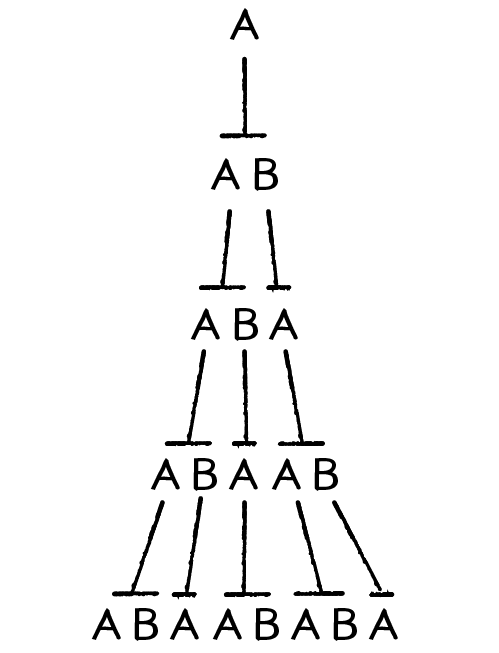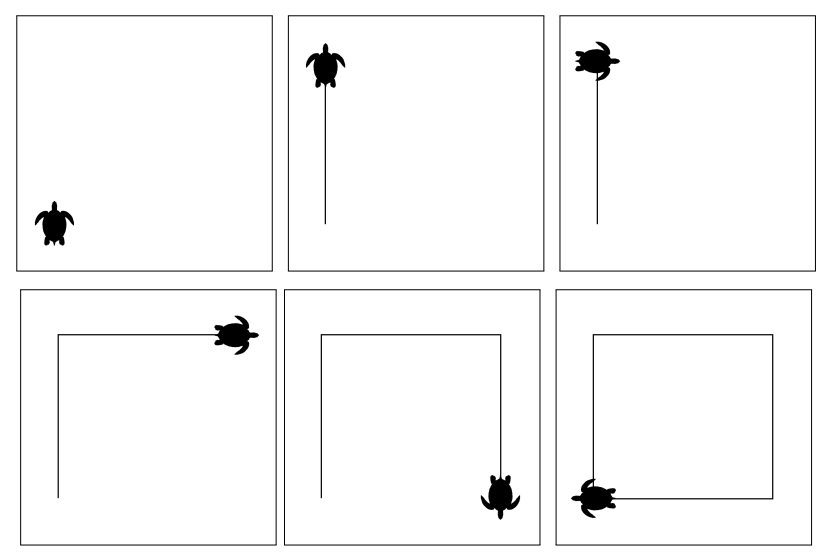In 1968, the Hungarian botanist Aristid Lindenmayer developed a grammar-based system to model and simulate the growth patterns of plants. Originally the L-Systems (short term for Lindenmayer systems) were devised to provide a formal description of the development of simple multicellular organisms while illustrating the neighborhood relationships between plant cells. Later on, this system was extended to describe higher plants and complex branching structures.

The realistic modeling of plant growth is of very high value to biology, physics and also for computer graphics (e.g. video games are using and still working on it to improve plants/forests rendering).

We will see how it works and use them to generate all kind of recursive fractal patterns. They are incredibly exciting as they provide a mechanism for keeping track of fractal structures that require complex and multi-faceted production rules.

## Composition

Let us quickly see the composition of the L-Systems, it involves four main components:

• Alphabet: Contains the valid symbols that can be managed. For example, we could say the alphabet is {‘F’, ‘X’, ‘Y’} meaning that any these three characters could be replaced given a rule.

• Constants: Symbols that do not get replaced. Most of the time the constants contains at least !, [, ], +, - (cf. Visual Interpretation) and are used for graphical instructions.

• Axiom: The axiom describes the initial state of the system (at iteration 0). It could be for instance “F”, “FX”, “F--F--F” or anything else.

• Rules: A rule is merely a transform taking a symbol as a parameter. The rules are applied successively to each symbol of the axiom and then over and over again at each iteration. If we take the rule “A —> AB”: whenever an “A” symbol is found in the current state, it is replaced with “AB.”

We have now everything to build a simple example; we will now study the Lindenmayer’s original system for modeling the growth of an algae.

## Example

• Alphabet: A, B
• Constants: none
• Axiom: A
• Rules: (A → AB), (B → A)

The system starts with “A” which is the axiom (iteration 0) and has two productions, one for A and one for B; have a look at the first five iterations:

 Iteration 0 Iteration 1 Iteration 2 Iteration 3 Iteration 4 A AB AB A AB A AB AB A AB AB AFor the first iteration we merely apply the first rule for A.
In the second iteration we have to apply the rules for A and B, etc. etc.
The derivations grow exponentially as a single letter often gets replaced by two or more letters in each iteration.

## Code

string LSystem(axiom, rules, depth)
{
string production;

// For each iteration:
for (auto level = 0; level < depth; ++level)
{
// Initialize production to empty string at each iteration
production = '';

// Iterate over each symbols/characters of the current axiom
for (auto symbol = axiom.begin(); symbol < axiom.end(); ++symbol)
{
// Transform it if a rule applies
if (rules.has(symbol))
production += rules.get(symbol);
// Add it without change otherwise
else
production += symbol;
}

// Update the axiom to its new content
axiom = production
}

return production;
} 

The recursive nature of the L-system rules leads to self-similarity and thereby fractal-like forms.
Now, let us see how those strings become descriptions of curves!

Recursive L-systems, like the one described above, often produce intricate patterns that are self-similar across multiple scales.

These patterns are almost impossible for a human to perceive directly from long strings of symbols. As with many types of data, a graphical representation may expose them.

The most typical graphical way of representing L-Systems is based on Turtle Interpretation (working as LOGO programming, an ancient  kid friendly way of teaching computer science with a Turtle), that draw by means of commanding a turtle in a Cartesian plane.

Imagine a turtle sitting on your computer screen to which you could issue a set of commands: move forward, turn left, turn right, draw a line, etc.

Now, we can associate each symbol to one command; the following dictionary is commonly used with L-Systems:

• F: Draw a line forward (demo default direction forwarding East)
• G: Move forward (without drawing a line)
• +: Turn left (of a particular angle)
• -: Turn right (of a particular angle)
• [: Push current location
• ]: Pop location

## Example

We will interpret the string F - F - F - F with a turning angle of 90◦ and starting facing north. While you can already imagine the final drawing, here is the Turtle making it:! It is time to produce a fractal !

We will create a Koch Triangle fractal (described above) step by step.

• Alphabet: F
• Angle Turn: 60°
• Constants: none
• Axiom: F
• Rules: F → F+F--F+F

We have seen here the simplest L-Systems to handle; however, more advanced systems exist and may be built. Here is the list of the different types that may be used:

• Deterministic context-free System or D0L-System: If there is precisely one production rule for each symbol, then the L-system is said to be deterministic. If each production rule refers only to a particular symbol and not to its neighbors, then the L-system is said to be context-free.

• Stochastic context-free System or S0L-Système If there are several production rules for a symbol that is chosen with a certain probability, then it is a stochastic L-system.

• Context Sensitive System, IL-System, or (k, l)-System: A context sensitive grammar looks not only at the symbol it is modifying, but the symbols appearing before and/or after it

• Parametric L-System: In a parametric grammar, each symbol in the alphabet has a parameter list associated with it. A symbol coupled with its parameter list is called a module, and a string in a parametric grammar is a series of modules.